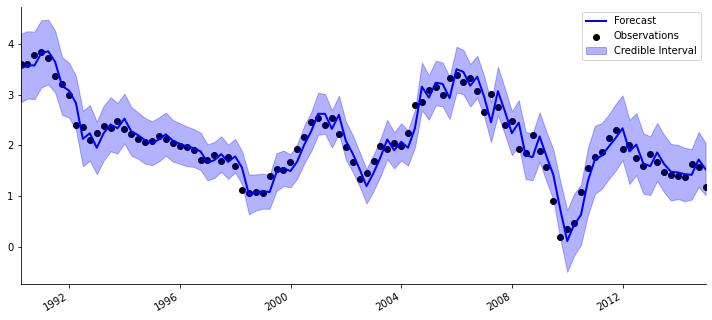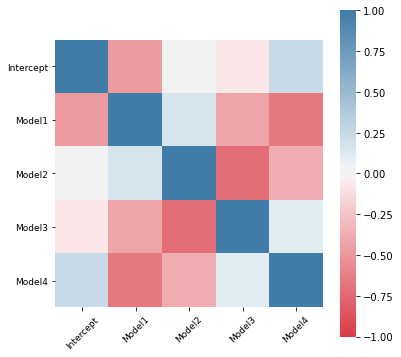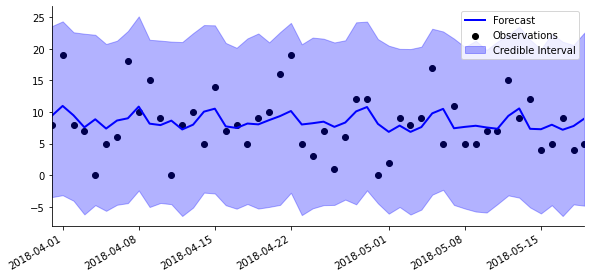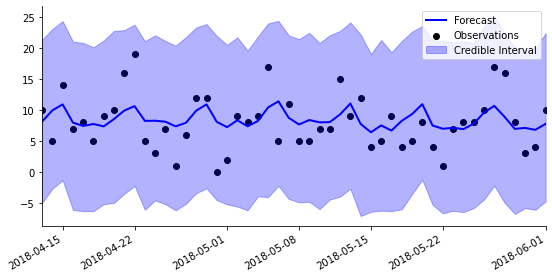A latent factor, which in PyBATS is a random variable used as a predictor for regression. By default, latent factors are described by a mean and a variance, but can also be integrated into a model through simulation, which is a more precise but slower process.

Latent factors are frequently used to model dependence among time series in a multivariate analysis. Several examples of doing this with a mean and variance are in Lavine, Cron, and West (2020), while examples using simulated values are given in Berry and West (2019).

One usage is for multiscale inference, where the time series have a hierarchical structure. For example, in retail sales you may observe both the total sales in a store and the sales of an individual item. The total sales in the store are more regular and predictable, so modeling them can produce smooth estimates of the day-of-week seasonality and the holiday effect at your store. These effects can be captured by a latent factor, and used as a predictor in a model of an individual item's sales, which are noisier and more unpredictable.

Let's say that the latent factor $\phi_t$ is created by model $\mathcal{M}_0$ of the time series $z_t$. The latent factor is used as a predictor in model $\mathcal{M}_i$ of the series $y_t$. The latent factor class describes $\phi_t$ through:

• A forecast mean and a variance, which is estimated before observing $z_t$, and is used to forecast $y_t$.
• An update mean and variance, which is estimated after observing $z_t$, and is used to update the state vector in $\mathcal{M}_i$ after observing $y_t$. Sometimes the latent factor is precisely known after observing $z_t$ (e.g. when the latent factor is $z_t$), and then the update variance is $0$.

Several common types of latent factors have been pre-defined in PyBATS:

1. seas_weekly_lf: The weekly seasonal effect latent factor.
2. hol_lf: The holiday effect latent factor
3. Y_lf: The observation latent factor. In this case, the forecast is simply the forecast mean and variance from $\mathcal{M}_0$, and the observed $z_t$ is used for updating.
4. pois_coef_lf, bern_coef_lf, and dlm_coef_lf: The coefficient (or state vector) latent factors for different types of DGLMs. These latent factors capture specified coefficients from $\mathcal{M}_0$.

Finally, the class multi_latent_factor concatenates multiple latent factors together when a model is using more than one latent factor as a predictor.

Simple examples of using latent factors are given below, and two more with a DCMM and a DBCM are provided in the examples folder.

## classlatent_factor[source]

latent_factor(mean={}, var={}, forecast_mean={}, forecast_var={}, forecast_cov={}, dates=[], forecast_dates=[], gen_fxn=None, gen_forecast_fxn=None, forecast_path=False, p=None, k=None)

## classmulti_latent_factor[source]

multi_latent_factor(latent_factors) :: latent_factor

The first latent factor example involves combining forecasts from different models. We have the forecast means and variances from 4 models that were used to predict quarterly US inflation from 1977 to 2014. One simple approach would be to use the 4 forecast means as predictors in a unified model. However, because we know the variance associated with each forecast, we can use a latent factor instead and account for the forecast uncertainty.

This example is inspired by Bayesian Predictive Synthesis, which is a sophisticated strategy for combining forecasts, and was first developed in McAlinn and West, 2016. This use of latent factors can be seen as an approximation to Bayesian Predictive Synthesis which allows for faster computation. The data in this example comes from that paper, and can be found here.

import numpy as np
import matplotlib.pyplot as plt

from pybats.define_models import define_dglm
from pybats.analysis import analysis
from pybats.latent_factor import dlm_coef_lf
from pybats.point_forecast import mean
from pybats.loss_functions import MAPE
from pybats.plot import plot_corr, plot_data_forecast


We start by loading in the data, which includes quarterly US inflation as well as the forecast means and variances from the 4 models.

data = load_us_inflation_forecasts()
dates = data['Dates']


Next, we define the hyperparameters and create the latent factor.

In this situation we're using the forecast mean and variance for updating as well, so the mean and forecast mean are the same, as are the variance and forecast variance.

k = 1 #Forecast horizon: 1-quarter ahead
forecast_start = 50
forecast_end = 149
agents=[1,2,3,4]
nagents = len(agents)
Y = data['Inflation']

lf = latent_factor(
mean = {d:m.astype('float64') for d, m in zip(dates, list(data['model_mean'].values))},
var={d:np.diag(v).astype('float64') for d, v in zip(dates, list(data['model_var'].values))},
forecast_mean={d:[m.astype('float64')] for d, m in zip(dates, list(data['model_mean'].values))},
forecast_var={d:[np.diag(v).astype('float64')] for d,v in zip(dates, list(data['model_var'].values))},
forecast_dates=dates,
p = nagents,
k = k)


In this example we're choosing to manually define a model before running an analysis. The reason is that we have prior information about the coefficients, and want to incorporate that information into the analysis. Specifically, we're giving each of the 4 models an equal starting weight by setting their coefficient means equal to $1/4$, and setting the intercept to $0$. The key parameters are:

• $a_0$, the prior mean of the coefficients
• $R_0$, the prior covariance matrix of the coefficients
• $s_0$, the prior observation variance in the normal dlm
• $n_0$, the prior weight given to $s_0$, so the higher $n_0$ is the more confident the model is in $s_0$.
kwargs = {'a0':np.concatenate([np.zeros(1), np.array([1/nagents]*nagents)]).reshape(-1,1),
'R0':np.identity(nagents+1) / 0.99,
's0':0.01,
'n0':5*0.99,
'deltrend': 0.99,
'dellf':0.99,
'delVar':0.99
}

mod_prior = define_dglm(Y=Y, X=None,
family='normal',
seasPeriods=[], seasHarmComponents=[[]],
nlf=4,
**kwargs)


The analysis is run as normal, but with two extra arguments:

• The latent factor, latent_factor=lf
• The initialized model, model_prior=mod_prior.

Setting prior_length=0 means that we aren't using any of the observations in $Y$ to set our prior.

prior_length = 0

samples, mod = analysis(Y, X = None, family = "normal",
prior_length = prior_length, k = 1, ntrend = 1, nsamps = 5000,
forecast_start = forecast_start, forecast_end = forecast_end,
model_prior = mod_prior,
latent_factor = lf,
dates = dates,
ret = ['forecast', 'model'],
**kwargs)

beginning forecasting


To evaluate the model, we can look at the forecast accuracy in terms of mean absolute percent error (MAPE):

forecast = mean(samples)
np.round(MAPE(Y[forecast_start: forecast_end+1], forecast), 3)

12.683

And plot the $1-$quarter ahead forecasts. There is a clear 'lagged' pattern in the forecasts, in which the forecast is close to the previous observation. This is because the previous observation is a very important predictor in the 4 models that we are averaging together.

fig, ax = plt.subplots(figsize=(10,5))
plot_data_forecast(fig, ax,
Y[forecast_start:forecast_end+1],
forecast, samples,
dates[forecast_start:forecast_end+1],
linewidth = 2);We can also look at the correlation matrix of our coefficients, telling us which ones are positively and negatively correlated. Typically, highly correlated variables have negatively correlated coefficients.

D = np.sqrt(mod.R.diagonal()).reshape(-1,1)
corr = mod.R/D/D.T

fig, ax = plt.subplots(figsize=(6,6))
plot_corr(fig, ax, corr=corr, labels = ['Intercept', 'Model1', 'Model2', 'Model3', 'Model4']);## Common Latent Factors

### Y (outcome) latent factor

#### Y_fxn[source]

Y_fxn(date, mod, Y, **kwargs)

#### Y_forecast_fxn[source]

Y_forecast_fxn(date, mod, X, k, nsamps, horizons, forecast_path=False, **kwargs)

### Weekly seasonal latent factor

#### seas_weekly_fxn[source]

seas_weekly_fxn(date, mod, **kwargs)

#### seas_weekly_forecast_fxn[source]

seas_weekly_forecast_fxn(date, mod, k, horizons, forecast_path=False, **kwargs)

### Holiday latent factor

#### hol_fxn[source]

hol_fxn(date, mod, X, **kwargs)

#### hol_forecast_fxn[source]

hol_forecast_fxn(date, mod, X, k, horizons, forecast_path=False, **kwargs)

This example demonstrates how to use the observation, seasonal, and holiday latent factors. The dataset contains simulated retail sales. totaldata are the daily total store sales, and data are the daily sales of a single item. We will fit a normal DLM to the log of total sales, and use it to learn the desired latent factors. These latent factors will then become predictors the sales of the individual item. This is considered a multiscale analysis because of the hierarchical relationship between the two time series.

First we load in the data:

import matplotlib.pyplot as plt

from pybats.plot import plot_data_forecast
from pybats.latent_factor import Y_lf, seas_weekly_lf, hol_lf, multi_latent_factor
from pybats.analysis import analysis
from pandas.tseries.holiday import USFederalHolidayCalendar
from pybats.point_forecast import median

totaldata, data = data.values()
totaldata['Y'] = np.log(totaldata['Y'] + 1)


Y X
2014-06-01 5.613128 -0.133124
2014-06-02 5.602119 -0.070102
2014-06-03 5.831882 1.020901
2014-06-04 5.393628 2.219605
2014-06-05 5.267858 -0.970556

And then run a standard analysis for the normal DLM. We will send in the list of empty new_latent_factors that will be populated by model. We also need to specify ret so that latent factors are returned from this analysis.

Defining the forecast dates is important, even though we aren't trying to forecast the total sales. We need to forecast the latent factor so it can be used as a predictor in the individual item forecast.

k = 14 # Number of days ahead that we will forecast
prior_length = 21
holidays=USFederalHolidayCalendar.rules

# Define forecast range for final year of data
T = len(totaldata)
forecast_end_date = totaldata.index[-k]
forecast_start_date = forecast_end_date - pd.DateOffset(days=365)

# Get multiscale signal (a latent factor) from higher level log-normal model
latent_factors = analysis(totaldata['Y'].values, totaldata['X'].values, k,
forecast_start_date, forecast_end_date,
family="normal", dates=totaldata.index,
seasPeriods=, seasHarmComponents=[[1,2,3]],
holidays=holidays,
ret=['new_latent_factors'],
new_latent_factors= [Y_lf, seas_weekly_lf, hol_lf],
prior_length=prior_length)

beginning forecasting


We now have a list of the 3 latent factors, which have been populated by the model. We can see their respective dimensions below:

[lf.p for lf in latent_factors]

[1, 7, 10]

The first latent factor is Y_lf, which stores both the actual total sales and the forecast total sales. The second is seas_weekly_lf, which is a $7-$dimensional vector. Each day, the element for today is populated with the estimated seasonal effect, while the other $6$ values are set to $0$. Finally, we defined $10$ holidays. This latent factor is only populated on the holidays themselves, so it is mostly just $0$.

We can get the update mean for each latent factor at any given date:

latent_factor_names = ['Total Sales', 'Weekly Seasonal', 'Holiday']
for lf, name in zip(latent_factors, latent_factor_names):
print(name + ' mean:', lf.get_lf(forecast_start_date).round(2))

Total Sales mean: 5.56
Weekly Seasonal mean: [ 0.    0.    0.    0.   -0.06  0.    0.  ]
Holiday mean: [0. 0. 0. 0. 0. 0. 0. 0. 0. 0.]


And also the forecast mean for any date in the forecast window. Here are the $1:14$ day ahead forecasts for the total sales:

print(latent_factor_names + ' forecast mean: ', np.array(latent_factors.get_lf_forecast(forecast_start_date)).round(2))

Total Sales forecast mean:  [5.42 5.65 5.76 5.56 5.39 5.36 5.35 5.43 5.63 5.8  5.13 5.38 5.36 5.35]


The $14-$day ahead forecast of the seasonal effects makes it clear how the latent factor works:

print(latent_factor_names + ' forecast mean: ')
print(np.array(latent_factors.get_lf_forecast(forecast_start_date)).round(2))

Weekly Seasonal forecast mean:
[[ 0.    0.    0.    0.   -0.06  0.    0.  ]
[ 0.    0.    0.    0.    0.    0.15  0.  ]
[ 0.    0.    0.    0.    0.    0.    0.29]
[ 0.03  0.    0.    0.    0.    0.    0.  ]
[ 0.   -0.12  0.    0.    0.    0.    0.  ]
[ 0.    0.   -0.13  0.    0.    0.    0.  ]
[ 0.    0.    0.   -0.15  0.    0.    0.  ]
[ 0.    0.    0.    0.   -0.06  0.    0.  ]
[ 0.    0.    0.    0.    0.    0.15  0.  ]
[ 0.    0.    0.    0.    0.    0.    0.29]
[ 0.03  0.    0.    0.    0.    0.    0.  ]
[ 0.   -0.12  0.    0.    0.    0.    0.  ]
[ 0.    0.   -0.13  0.    0.    0.    0.  ]
[ 0.    0.    0.   -0.15  0.    0.    0.  ]]


The same output can be created for the holiday latent factor, but it will be all $0$'s except when there is a holiday in the next $14$ days.

Now let's combine these latent factors into a single multi_latent_factor, and put them to use in an analysis of the individual item sales. First we create the multi latent factor:

multi_lf = multi_latent_factor(latent_factors[:3])
print('Total dimension :', multi_lf.p)

Total dimension : 18


And then we run a standard analysis, while passing in the multi_lf as our latent factor. We're setting the discount factor on the latent factor component as dellf=1, which means no discounting. This is because the weekly seasonal pattern and holidays are $0$ so often. Another option would be to define dellf as an $18-$dimensional vector, with a separate discount factor for each element of the latent factor.

nsamps = 1000

mod, forecast_samples = analysis(data['Y'].values, data['X'].values, k,
forecast_start_date, forecast_end_date,
nsamps=nsamps,
latent_factor = multi_lf,
dates=data.index,
prior_length = prior_length,
s0=5, dellf=1)

forecast = median(forecast_samples)

beginning forecasting


Looking at the forecasts, we can see a weekly seasonal pattern come through from the latent factors:

horizon = 1
plot_length = 50
fig, ax = plt.subplots(figsize=(8,4))
start_date = forecast_end_date + pd.DateOffset(horizon - plot_length)
end_date = forecast_end_date + pd.DateOffset(horizon - 1)
ax = plot_data_forecast(fig, ax,
data.loc[start_date:end_date].Y,
forecast[-plot_length:,horizon - 1],
forecast_samples[:,-plot_length:,horizon - 1],
data.loc[start_date:end_date].index,
linewidth = 2)And the same is true in the 14-day ahead forecast:

horizon = 14
plot_length = 50
fig, ax = plt.subplots(figsize=(8,4))
start_date = forecast_end_date + pd.DateOffset(horizon - plot_length)
end_date = forecast_end_date + pd.DateOffset(horizon - 1)
ax = plot_data_forecast(fig, ax,
data.loc[start_date:end_date].Y,
forecast[-plot_length:,horizon - 1],
forecast_samples[:,-plot_length:,horizon - 1],
data.loc[start_date:end_date].index,
linewidth = 2)### Model coefficient latent factors

#### pois_coef_fxn[source]

pois_coef_fxn(date, mod, idx=None, **kwargs)

#### pois_coef_forecast_fxn[source]

pois_coef_forecast_fxn(date, mod, k, idx=None, **kwargs)

#### bern_coef_fxn[source]

bern_coef_fxn(date, mod, idx=None, **kwargs)

#### bern_coef_forecast_fxn[source]

bern_coef_forecast_fxn(date, mod, k, idx=None, **kwargs)

#### dlm_coef_fxn[source]

dlm_coef_fxn(date, mod, idx=None, **kwargs)

#### dlm_coef_forecast_fxn[source]

dlm_coef_forecast_fxn(date, mod, k, idx=None, forecast_path=False, **kwargs)

The model coefficient latent factors are easy to understand. They store the state vector means and variances over time. If we simply wanted to plot the trajectory of a coefficient after an analysis, we don't need to use a latent factor. This is available in analysis by including 'model_coef' in the list of items to return.

However, the latent factor can be useful for forecasting in two related models. For example, if there are two time series with very related predictors, then the coefficient from one model, multiplied by the predictor, may be a strong latent factor in another model.

### Helper Functions

#### merge_fxn[source]

merge_fxn(date, latent_factors, **kwargs)

#### merge_forecast_fxn[source]

merge_forecast_fxn(date, latent_factors, **kwargs)

#### merge_latent_factors[source]

merge_latent_factors(latent_factors)

merge_latent_factors is used to average together a set of latent factors. An example usage: There are a set of models, all with the same regression predictor. The coefficient latent factor is saved from an analysis. This function will produce a new latent factor which is the precision-weighted average effect from that coefficient.

#### merge_lf_with_predictor[source]

merge_lf_with_predictor(latent_factor, X, X_dates)

The function merge_lf_with_predictor multiplies a latent factor by a known predictor. This is useful in combination with the coefficient latent factors. To save a regression effect, the coefficient latent factor can store the dynamic coefficient from an analysis. Then, that latent factor can be multiplied by the predictor, to produce the full regression effect.